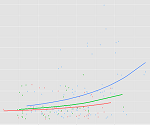# How to Combine Complicated Models with Tricky Effects

by

Interpret interactions in a logistic regression?This is where statistical analysis starts to feel really hard. You’re combining two difficult issues into one.

You’re dealing with both a complicated modeling technique at Stage 3 (survival analysis, logistic regression, multilevel modeling) and tricky effects in the model (dummy coding, interactions, and quadratic terms).

The only way to figure it all out in a situation like that is to break it down into parts.  Trying to understand all those complicated parts together is a recipe for disaster.

But if you can do linear regression, each part is just one step up in complexity.  Take one step at a time.

### The Type of Model Reflects the Distribution of the Response Variable and the Design

Most statistical models are extensions of linear models (aka. the General Linear Model).  The extensions deal with linear model assumptions that your data don’t meet.

Since most assumptions are about the residuals, whose distribution reflects the measurement of the response variable, these extensions are all for response variables that don’t fit the general linear model.  Our examples above are necessary for censored time-to-event, categorical, and clustered response variables.

The other regression models mostly use maximum likelihood estimation, which requires different measures of model fit.

They also often use link functions, making interpretation of coefficients harder. (Remember, coefficients measure the relationship between the predictor and response, so the link function on the response always affects the scaling of the coefficients).

So the first thing you need to work on is how to implement, interpret coefficients, and understand model fit in the model you’re using.  Don’t worry yet about the tricky effects until you’ve mastered the model you’re using with simple, continuous predictors.

### The Tricky Effects are About the Predictor Variables

The nice thing about all these models is that the fixed part of the right hand side of the equation are all the same. This is the part with the regression coefficients and X variables. This part of the model measures and tests the effects you’re interested in.

A dummy variable is a dummy variable in every kind of model.

The random parts will differ, but they’re not directly involved with the effects of predictor variables. (In most models, the only random part is the residuals).

All those tricky effects occur when the predictors aren’t the textbook-perfect numerical variables you used in regression class.  Examples include dummy variables for categorical predictors, interactions and quadratic terms that are the products of two predictors, centered or rescaled predictor variables.

So once you learn how and when to use, and how to interpret any tricky effect in a linear model, you can use it in any type of model.

I generally recommend mastering these effects in the context of linear models. Even if that’s not your immediate need.  Because learning how to work with and interpret the effects of dummy variables is a lot harder if you’re doing it in terms of odd ratios than in terms of comparing means.

So the next time you need to figure out a combination of a tricky effect and a complicated model, break it down first.  Simplify your model as much as you can as you master each part.

This might mean you run some simple preliminary models that you never could publish. That’s okay. Taking the time to understand each part will be worth it.Interpreting Linear Regression Coefficients: A Walk Through Output
Learn the approach for understanding coefficients in that regression as we walk through output of a model that includes numerical and categorical predictors and an interaction.

### Related PostsIngo Peters

This short article on tricky effects was very useful for me and clearified my interpretation of a cox regression with a quadratic term. Thanks!HdS

Hello,
Part of the problem is that there are not many publications regarding “tricky” problems. I wanted to do a interaction in a cox regression and where not able to find any good adivce for their interpretation.Karen